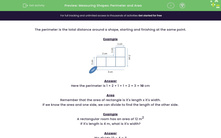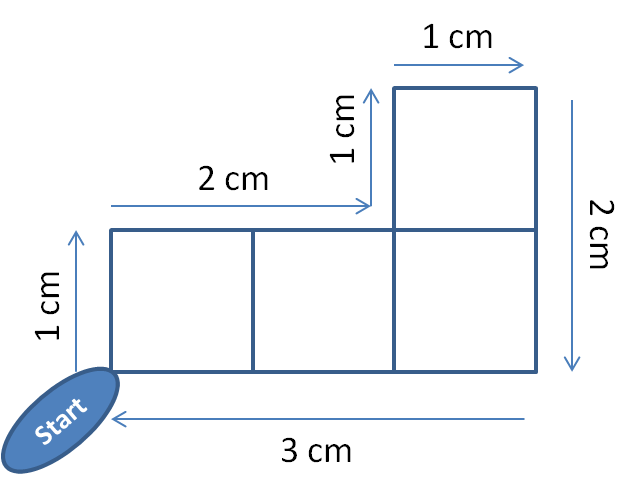# Calculate Areas and Perimeters of Rectangles

In this worksheet, students will answer questions on area and perimeter.Key stage:  KS 2

Curriculum topic:   Measurement

Curriculum subtopic:   Measure and Calculate Shapes

Popular topics:   Area worksheets, Shape worksheets

Difficulty level:#### Worksheet Overview

The perimeter is the total distance around a shape, starting and finishing at the same point.

ExampleHere, the perimeter is 1 + 2 + 1 + 1 + 2 + 3 = 10 cm

The area of a rectangle is length x width.

Example

If we have a rectangle with a width of 4 m and a length of 6 m:

The area will be 4 m x 6 m = 24 m2Calculate a missing length

If we know the area and one side, we can divide to find the length of the other side.

Example

A rectangular room has an area of 12 m2

If its length is 4 m, what is its width?

We divide 12 ÷ 4 = 3

So the width = 3 m

How do you feel about that one?Let's have a go at some questions now.

### What is EdPlace?

We're your National Curriculum aligned online education content provider helping each child succeed in English, maths and science from year 1 to GCSE. With an EdPlace account you’ll be able to track and measure progress, helping each child achieve their best. We build confidence and attainment by personalising each child’s learning at a level that suits them.

Get started